# Sample Data: Sea Anemones

Locations of sea anemones (marine predatory animals, often quite colourful ), annotated with diameter marks

## Details

Locations of sea anemones (marine predatory animals, often quite colourful ) in the observation region Rectangle[{0., 0.}, {280., 180.}], annotated with diameter marks.

## Examples

### Basic Examples (2)

Retrieve the data:

 In:=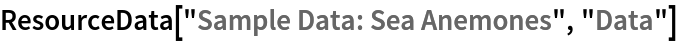Out=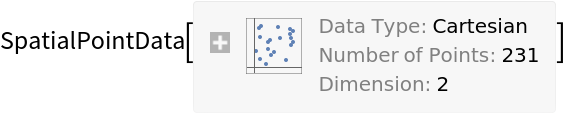Summary of the spatial point data:

 In:=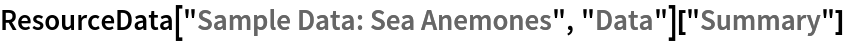Out=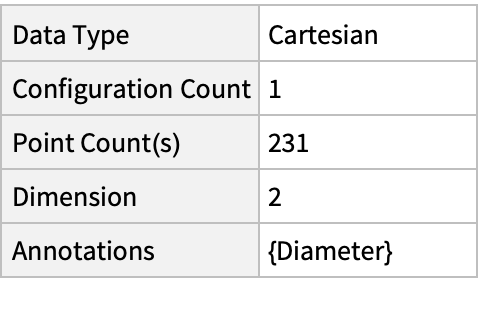### Visualizations (3)

Plot the spatial point data:

 In:=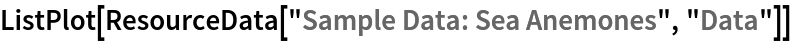Out=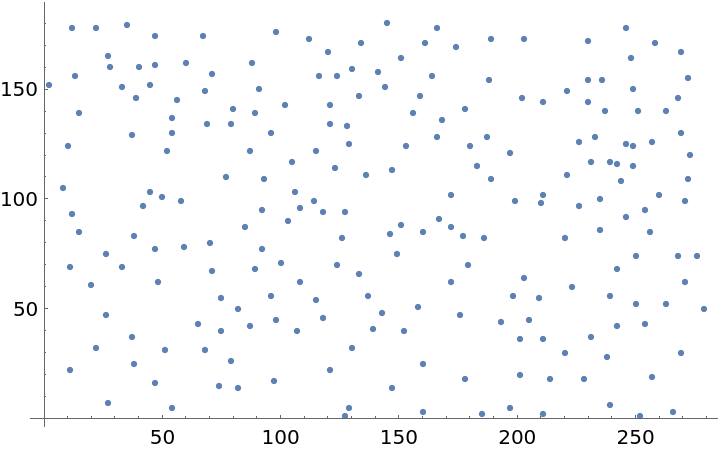Visualize the points with annotations:

 In:=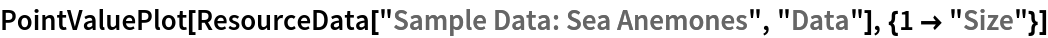Out=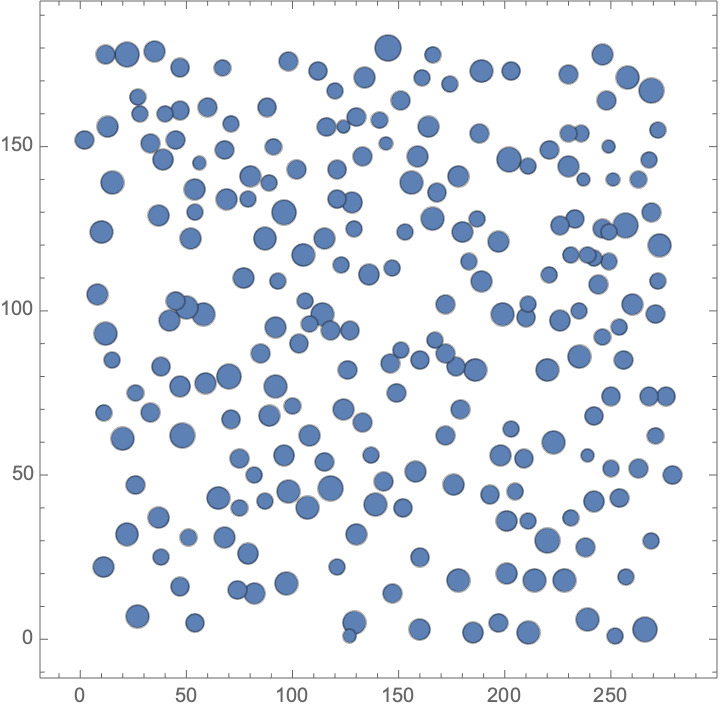Visualize the smooth point density:

 In:=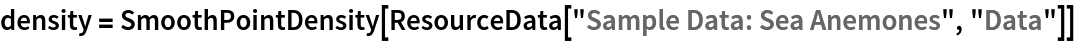Out=In:=Out=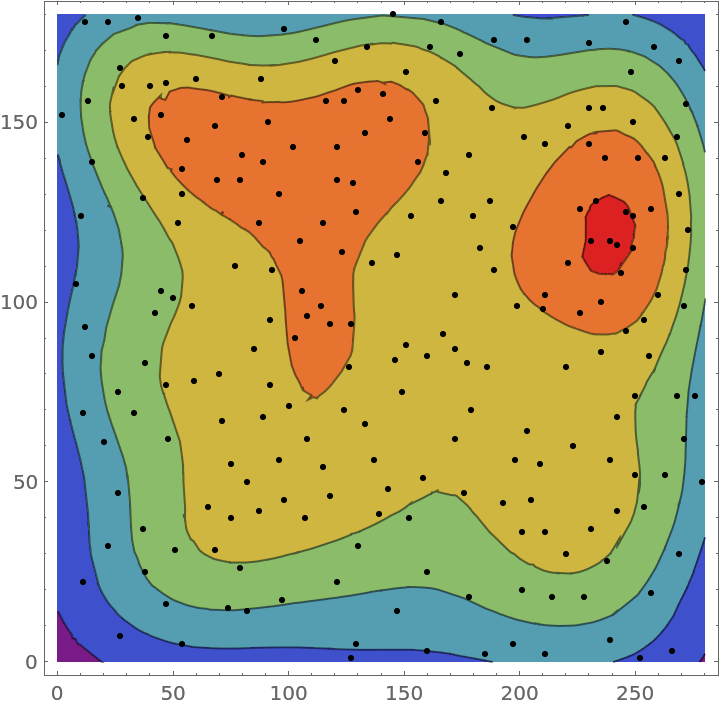### Analysis (4)

Compute probability of finding a point within given radius of an existing point - NearestNeighborG is the CDF of the nearest neighbor distribution:

 In:=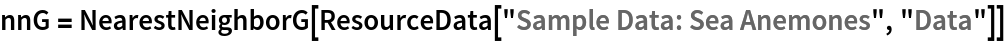Out=In:=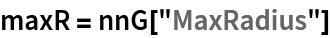Out=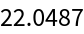In:=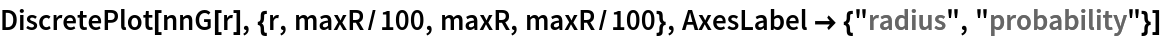Out=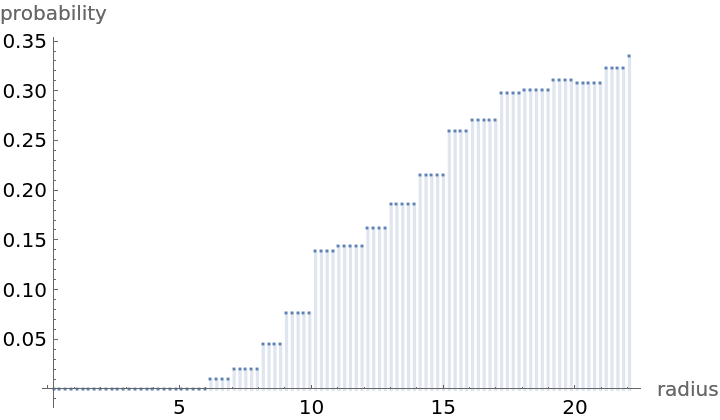Mean distance between a typical point and its nearest neighbor (for positive support distribution can be approximated via a Riemann sum of 1-CDF):

 In:=In:=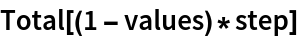Out=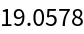Test for complete spacial randomness:

 In:=Out=Fit a Poisson point process to data:

 In:=Out=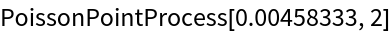Gosia Konwerska, "Sample Data: Sea Anemones" from the Wolfram Data Repository (2021)